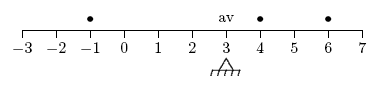﻿ Average of Signed Numbers
AVERAGE OF SIGNED NUMBERS
by Dr. Carol JVF Burns (website creator)
Follow along with the highlighted text while you listen!

To average $\,N\,$ numbers, add them up and divide by $\,N\,$.

A convenient way to talk about $\,N\,$ numbers is to use subscript notation.
A subscript is a character (usually, a number or letter) that is written slightly below another character.
For example, when you look at $\,x_3\,$ (read as ‘$\,x$ sub $3\,$’), the number $\,3\,$ is a subscript.
When you look at $\,y_b\,$ (read as ‘$\,y$ sub $b\,$’), the letter $\,b\,$ is a subscript.

In subscript notation, we can let $\,x_1\,$ denote the first number, $\,x_2\,$ denote the second number, and so on.

AVERAGE OF $\,N\,$ NUMBERS
Let $\,x_1, x_2, x_3, \ldots, x_N\,$ denote $\,N\,$ numbers.
Then, the average of these $\,N\,$ numbers is:
$$\frac{x_1 + x_2 + x_3 + \cdots + x_N}{N}$$

When more than two numbers are averaged, the concept of balancing point becomes the central idea.

To illustrate the idea, consider finding the average of three numbers: $\,-1\,$, $\,4\,$, and $\,6\,$.
Put equal-weight pebbles at locations $\,-1\,$, $\,4\,$ and $\,6\,$ on a number line.
If you think of the number line as a see-saw from a childhood playground,
the support must be placed at the average, for perfect balance!

$$\text{average } = \frac{-1 + 4 + 6}{3} = \frac{9}{3} = 3$$It is clear from the ‘balancing point’ interpretation of the average that
the average of numbers always lies between the greatest number (the one farthest to the right)
and the least number (the one farthest to the left).

EXAMPLE:
Question: Find the average of $\,5\,$, $\,6\,$, and $\,-2\,$.
Solution:
$\displaystyle\text{average} = \frac{5 + 6 + (-2)}{3} = \frac{9}{3} = 3$
Master the ideas from this section

When you're done practicing, move on to:
Identifying Place Values